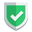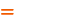我们相信：世界是美好的，你是我也是。平行空间的世界里面，不同版本的生活也在继续...

`python`的思路不是一般的清奇，各种语法思路都和其它编程语言不一致，具有较大的区别。那么，`python`语言里面是如何实现类的继承的呢？又是如何判断类的继承关系的呢？带着这些疑问来查看本文的内容吧。

## 普通的类

`python`中普通的类写法如下：

``````class University:
def __init__(self,name):
self.name = name
def introduce(self):
print("这是"+self.name)

U = University("北京大学")
U.introduce()
print('"U" IS university?', isinstance(U, University))``````
• 在类名后面没有括号。
• 类初始化，参数在`__init__`设置。
• 在类的方法里面，如果访问`self`的话，必须在定义的第一个参数里面写上`self`
• 调用类方法传递参数的时候，不用显示传递参数`self`
• 初始化类的时候，不用关键词`new`

## 继承类

``````class Person:
def __init__(self, name):
self.name = name
def introduce(self):
print("我是",self.name)
def introduce2(self): #Student子类调用就可以，但是自身调用报错
print("我是",self.name,"来自",self.school.name)
def introduce3(self):
print("我是将要被改写的方法")

class Student(Person):
def __init__(self,name,University):
super().__init__(name)
self.school = University
def introduce3(self):
print("我是",self.name,"同学,来自",self.school.name)``````

``````su = Student("苏南",University("北京大学"))
su.introduce()
su.introduce2()
su.introduce3()

pp = Person("苏南大叔")
pp.introduce()
pp.introduce3()
pp.introduce2()``````

## 网络流传代码

• 一般的类会继承于`(object)`。但是苏南大叔这边觉得没有啥好继承的时候，为啥要写？哈哈。
• 继承的子类在`__init__`里面，要`super(子类名,self)`，苏南大叔测试中写的代码是`super()`。目前的代码测试中，暂时没有啥问题。
``````class School(object):
def __init__(self):
pass

class University(School):
def __init__(self):
super(University,self).__init__()
self.name = name``````

## 判断是否属于某个类

``````print('"su" IS Student?', isinstance(su, Student), ">", type(su))
print('"pp" IS Student?', isinstance(pp, Student), ">", type(pp))
print('"su" IS Person?', isinstance(su, Person), ">", type(su))
print('"pp" IS Person?', isinstance(pp, Person), ">", type(pp))``````

• 考虑继承关系，就使用`isinstance`，更圆滑。
• 不考虑继承关系，就使用`type`，更精准。

## 综述

`python`的语法就是不走寻常路，这个类的继承语法，也是一如既往的清奇。如果本文对您有帮助，或者节约了您的时间，欢迎打赏瓶饮料，建立下友谊关系。本博客不欢迎：各种镜像采集行为。请尊重原创文章内容，转载请保留作者链接。【福利】 腾讯云最新爆款活动！1核2G云服务器首年50元！【源码】本文代码片段及相关软件，请点此获取更多信息【绝密】秘籍文章入口，仅传授于有缘之人python